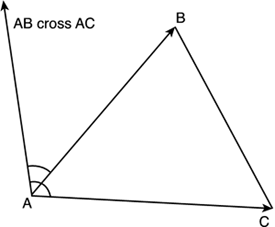# Prop Handling

One typical enhancement to our base animation system is the addition of props, which can range from weapons to clothes, or even vehicles. This feature adds richness to the game and has been key to the success of many popular titles. Luckily, accessories are relatively easy to program. All that is needed are some minor changes and a bit of math, and our characters will be able to carry (or be carried by) almost anything.

To begin with, we will need to add prop tags to our character. Basically, we need to know where each accessory attaches. The method I recommend is essentially the same used in the Quake III MD3 format, adding symbolic objects with no geometry value other than they help us store the orientation of the prop throughout the animation cycle. Then, we will consider the prop as a rigid object attached to the prop tag. This can easily be achieved by translating and rotating the prop to the attachment point.

The translation-rotation code can be implemented using two different strategies. If you are working on a frame-by-frame basis, the best choice is to use matrix math. On the other hand, if you are working with temporally distant keyframes, a more powerful operator such as quaternions will be a better match. For matrices, I suggest you build basis matrices for the orientation and use those to transform the prop to its definitive location. The approach is very similar to the one we used for skeletal animation:

1. Translate the pivot point to the origin.

2. Multiply by the basis matrix.

3. Translate to the final position.

And basis matrices are built easily from the tag's geometry. Assuming your tag is a triangle (which is what Quake used), you can extract two vectors from the triangle's sides: Segments AB and AC (see Figure 15.6) define them. Then, a cross product between them will give you the third vector. Storing them in column form will give you the basis:

##### Figure 15.6. Building a base to place props with.Right.x Up.x Front.x Pos.x Right.y Up.y Front.y Pos.y Right.z Up.z Front.z Pos.z 0 0 0 1

This matrix represents a transform, which, when fed with points in the form (x,y,z,1), will convert them to the coordinate system defined by the basis. In other words, if we transform each vertex in the prop using this matrix, the transformed vertices will be placed (and oriented) the way our marker depicts. Life could be easy and fun all the time, but not usually. Problems occur when no one expects them, and prop handling is a good example. To expose its problems, imagine that the sampling rate for the prop marker is lower than the rate at which you will be displaying animations. Or even worse, imagine that you want to use interpolation to smooth the animation and improve the results.

In both cases, your animation system would basically need to render something like frame number 3.765, which is definitely not 3, and not quite 4 either. We have seen how we can use interpolation to approximate the position of the vertices at any given point in time, integer or fractional. So how do we apply this to prop handling?

Quite clearly, our problem consists of generating a new transform basis for frame number 3.765. Unfortunately, this is easier said than done. How can we interpolate a matrix, or even worse, an orthonormal matrix? Remember that we need all column vectors to be normalized to have a modulus of 1. This is the normalization part of the equation. We also need the orthogonal part. The three vectors (up, front, right) must be perpendicular to each other, which effectively means the determinant must be 1.

At this point, I will make use of our initial assumption. Our sampling rate is high, so orientation differences from one frame to the next will be relatively small. As a result, we can safely do a direct interpolation of the basis matrices. This would be a deadly sin to a strict mathematician, but consider this:

• The bottom row (0 0 0 1) does not really affect the interpolation because it remains the same.

• The rightmost column represents the position, which can definitely be interpolated safely.

• The orientation 3x3 submatrix undergoes a minimal change if frames are close in time.

This process will introduce some artifacts because the interpolated matrix is not a transform basis in the strict sense. But the error incurred will be unnoticeable.

A more robust alternative would be to interpolate the two matrices and renormalize the three vectors (up, front, right) that make the 3x3 orientation submatrix. By doing this, we make sure we comply with the normalization condition of the basis. We can even interpolate front and right only, and recompute the up vector doing a cross product. This would increase the computational cost but help comply with the orthogonal condition.Core Techniques and Algorithms in Game Programming2003
ISBN: N/A
EAN: N/A
Year: 2004
Pages: 261